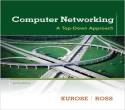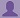SHARE
HELP

To start with, Sr2Jr’s first step is to reduce the expenses related to education. To achieve this goal Sr2Jr organized the textbook’s question and answers. Sr2Jr is community based and need your support to fill the question and answers. The question and answers posted will be available free of cost to all.# Computer Networking : A Top-down Approach

Authors:
James F. Kurose, Keith W. Ross
Exercise:
Problems
Chapter:
Computer Networks And The Internet
Edition:
6
ISBN:
9780132856201
Question:
3
 Previous Next

###Question

Consider an application that transmits data at a steady rate (for example, the sender generates an N-bit unit of data every k time units, where k is small and fixed). Also, when such an application starts, it will continue running for a relatively long period of time. Answer the following questions, briefly justifying your answer:

a. Would a packet-switched network or a circuit-switched network be more appropriate for this application? Why?

b. Suppose that a packet-switched network is used and the only traffic in this network comes from such applications as described above. Furthermore, assume that the sum of the application data rates is less than the capacities of each and every link. Is some form of congestion control needed? Why?

###Answer

a)

Circuit-switched network is the more appropriate for this application.

Reason: Fixed bandwidht and long sessions invloved.

b)

Consider that a packet-switched network is used and the only traffic in this network comes from such applications as described above. Furthermore, assume that the sum of the application data rates is less than the capacities of each and every link.In that time congestion control is needed. The reason is that sufficient bandwidth of the link is enabled to completed the task of application.

###R Pandya

Assuming the sum of the application data rates is less than the capacities of each and every link, no congestion control is needed even in wost case scenario.

Post the discussion to improve the above solution.